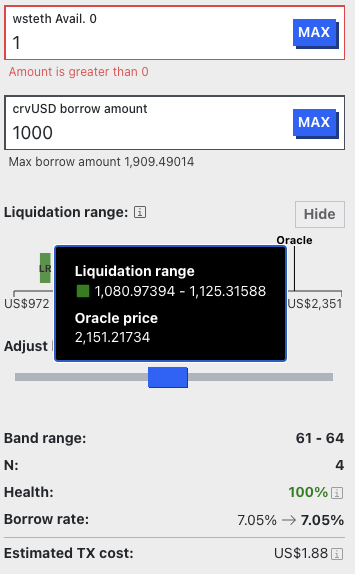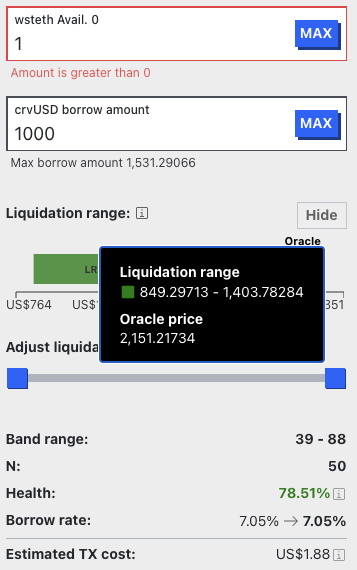# User guide of crvUSD and introduction of rate & LLAMMA

By 0xmc,0xstan@0xreviews, paco

## The Summary

This article discusses the design and concepts behind the stablecoin crvUSD, including the lending-liquidating amm algorithm (LLAMMA), PegKeeper, and Monetary Policy. The main idea behind LLAMMA is to use a special-purpose AMM to replace liquidations. The article explains how LLAMMA works, including the role of arbitrageurs in buying low and selling high. The article also discusses the Controller and Monetary Policy, including how the debt fraction and PegKeeper work. Risks associated with liquidation are covered, including the unrecoverable loss experienced during soft-liquidation. Strategies for minimizing risk and maximizing borrowing are also discussed.

## 0. overview

The stablecoin design has a few concepts: lending-liquidating amm algorithm (LLAMMA), PegKeeper, and Monetary Policy are the most important ones. But the main idea is in LLAMMA: replacing liquidations with a special-purpose AMM.

The basic idea of LLAMMA is to automatically buy low and sell high through the behaviors of arbitrageurs interested in a well-designed price difference between P_AMM and P_oracle.

This article is also a pre-knowledge article for the subsequent release of the analysis report on crvUSD arbitrage behavior. We're going to introduce crvUSD following the schematic from crvUSD whitepaper.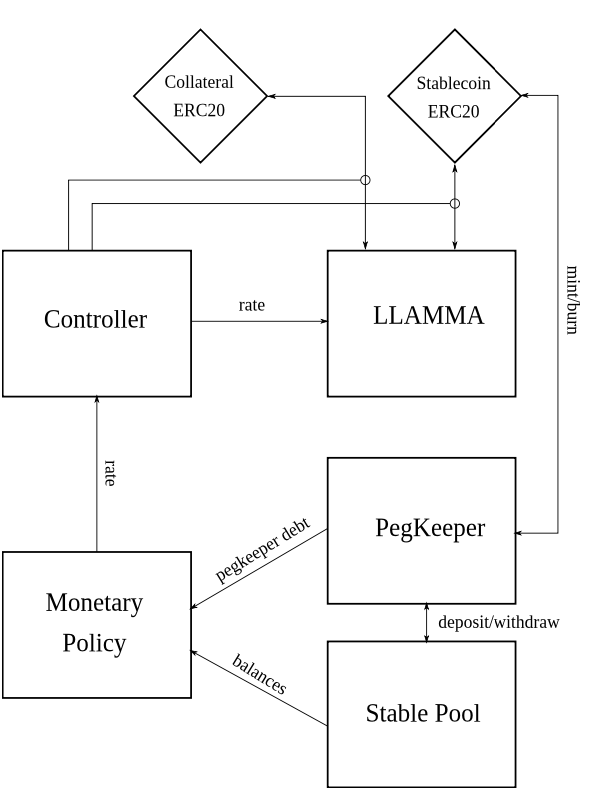## 1. LLAMMA and hard-liquidation

### 1.1 Why we need LLAMMA

In a typical automated market maker (AMM) like Uniswap V3, a liquidity provider (LP) puts single ETH into the pool. The ETH will be converted to USDT when the price rises to a certain point and will be converted back to ETH when it falls to the corresponding price.

However, in LLAMMA, we want the opposite to happen. When the price falls to a certain point, the position will change to USDT to no longer decrease. When the price rises, we hold all ETH positions, so LLAMMA is suitable for settlement in the soft-liquidation of lending.

This process requires the introduction of arbitrageurs to sell the ETH we want to buy when the ETH price rises and sell the ETH we want to sell when the ETH falls. LLAMMA pool quotes need to be adjusted to provide arbitrageurs with trading incentives. When the ETH price rises, the prices in the LLAMMA pool should be higher, making it more willing for outsiders to sell ETH to the pool. When the ETH falls, the ETH price should be lower, which allows outsiders to arbitrage in the pool, with price increases converting to ETH and price decreases converting to USDT.

Therefore, we need to make the liquidity and virtual reserves in regular AMMs like Uniswap V3 functions of P_oracle to adjust the price according to P_oracle. Finding such a function will allow us to obtain a very suitable AMM for settlement, namely LLAMMA.

### 1.2 LLAMMA introduction

1. Bob deposited 1ETH at \$2000 and added 1ETH to the LLAMMA pool, and chose N=5. His 1ETH will be distributed from price 1900 to 1881(5 bands)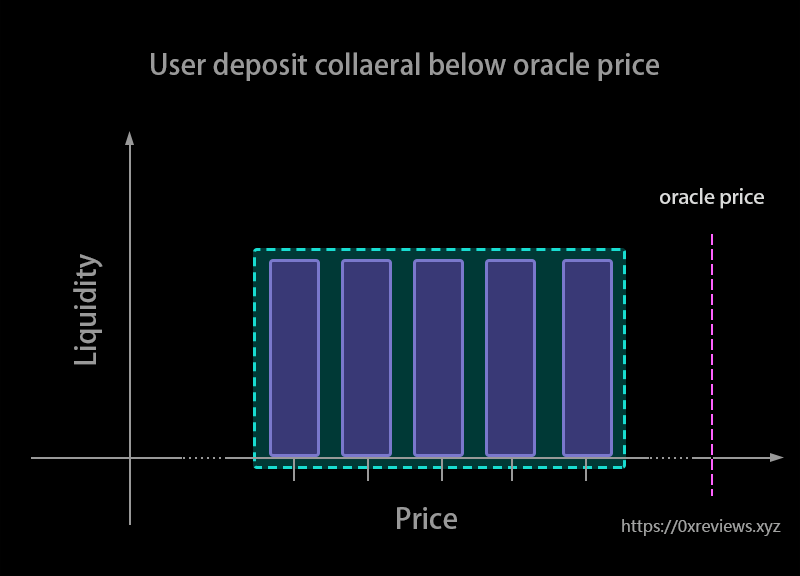2. When AMM Price goes down and lower than 1900, liquidation happens. Oracle's price is 1899 between 1990 to 1980, while LLAMMA's price is designed to be 1897 lower than 1899. The profit rate of the arbitrageurs‘ swap in this band will be (1899-1897) /1899 = 0.1%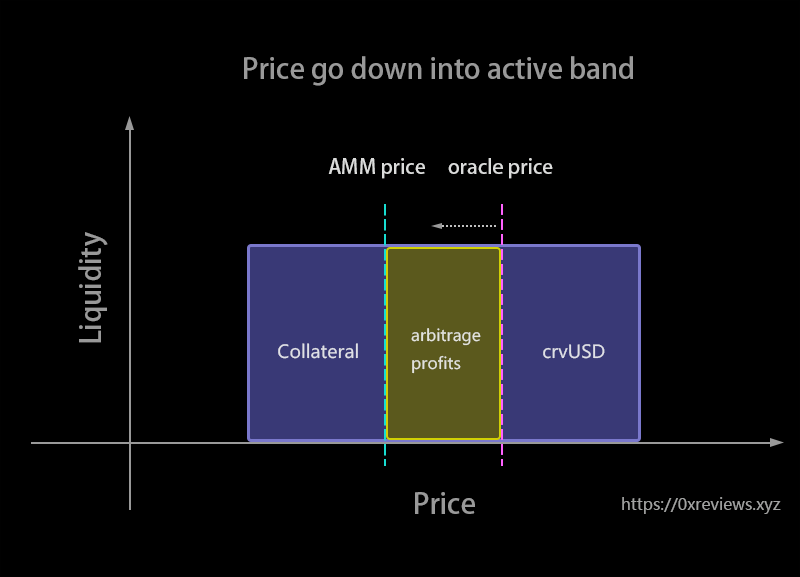3. Oracle price is 1880 out of 1900-1881. Bob’s ETH will all be transformed into crvUSD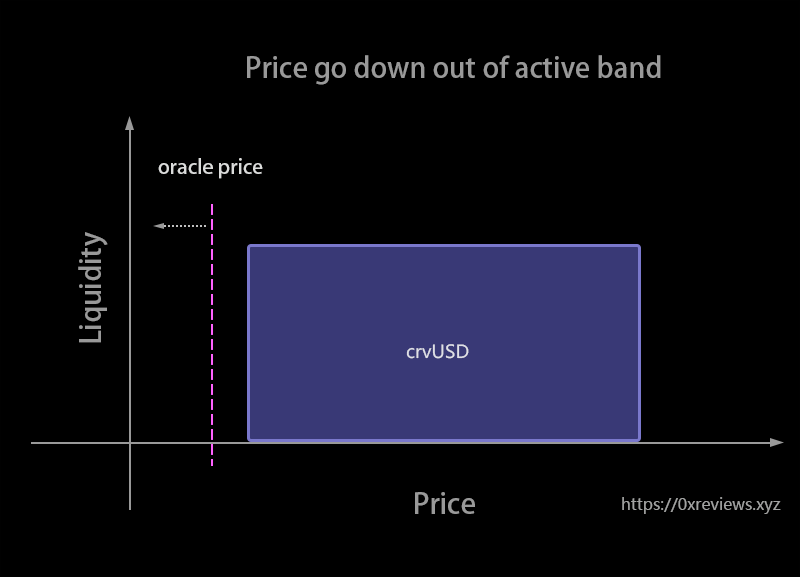4. When AMM Price goes up and higher than 1881, the de-liquidation happens. Oracle price is 1882 between 1881 to 1985 while LLAMMA price is designed to be 1883 higher than 1899. Bob’s crvUSD is transforming into ETH. The profit rate of the arbitrageurs’ swap in this band will be (1883-1881) /1899 = 0.1%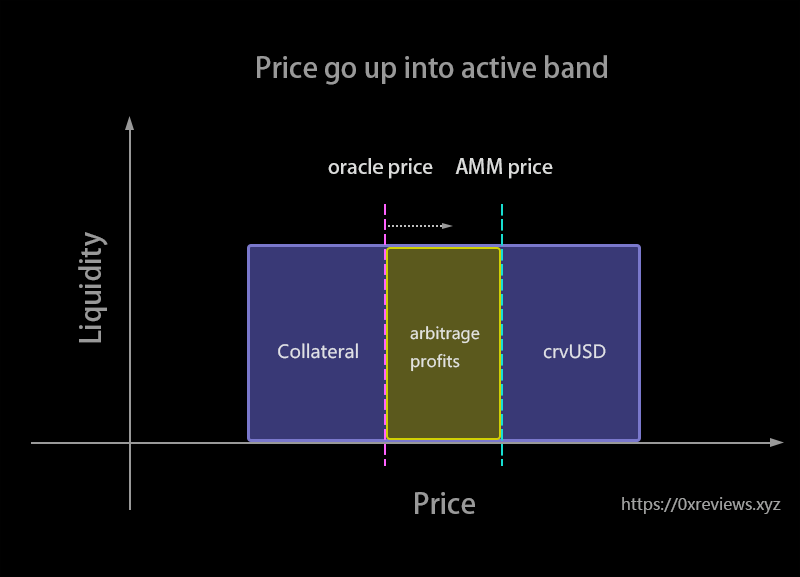5. Oracle price is 1991 out of 1900-1881. Bob’s crvUSD will all be transformed into ETH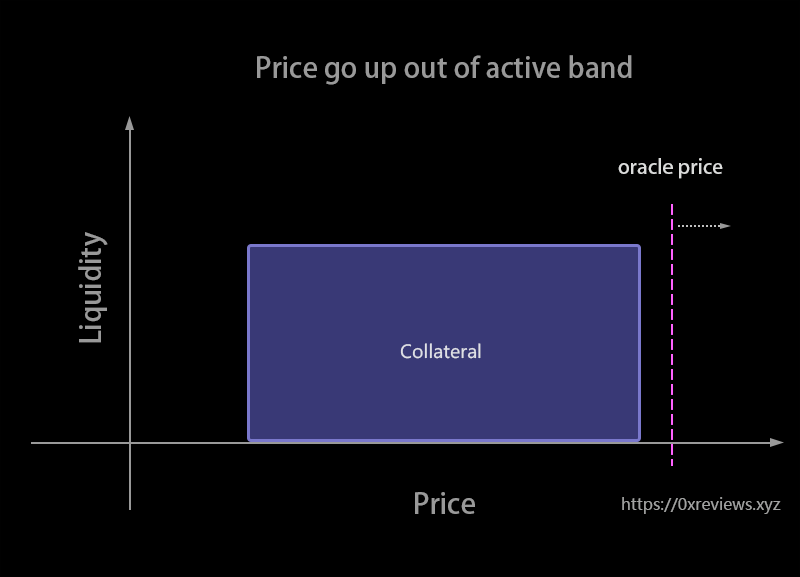## 2. Controller

The Controller provides the interface for user interaction, and internally interfaces with LLAMMA to manage the deposit and withdrawal of user assets.

When a user creates a debt by depositing collateral, they must specify the following parameters:

• The amount of ETH collateral
• The size of the created crvUSD debt
• The number of consecutive bands within which the ETH will be deposited into LLAMMA

The minimum number of bands for the third parameter is 4, and the maximum is 50. The Controller will calculate the bands that the user needs to deposit based on these three parameters, and will deposit the user's ETH into those bands (which are necessarily out of price, so adding liquidity is adding ETH as a single currency). At the same time, crvUSD will be sent to the user, and the user's debt will be equal to the amount of minted crvUSD.

The Controller also has functions for increasing collateral, repaying debt, withdrawing collateral, performing force liquidations, and maintaining loan interest rates.

Mathematical Principle:

the loan_discount_rate r = 9%, A = 100. Loan-to-Value (LTV) Ratio rate is mainly related to N. The smaller N is, the larger LTV will be.

$LTV = \frac{(1 - r)}{N} \cdot \sqrt{(A-1)A} \cdot (1-(\frac{A-1}{A})^N)$

MAX crvUSD borrow amount :

$MAXcrvUSD\ borrow =LTV\_max * Base price*((A-1)/A)^{active\_band}$

Health:

$health\_factor = asset\_value/debt -hard\_liquidation\_discount -100\%$

The hard_liquidation_discount R is 6%. When health_factor is below 1, the hard-liquidation happens.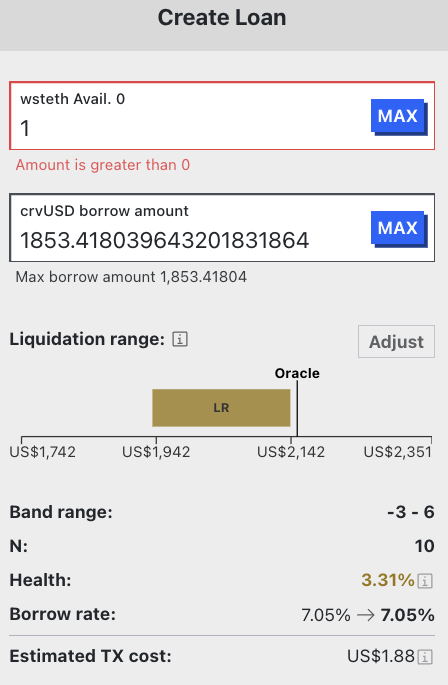## 3. Monetary Policy

Debt_Fraction: $D_F$

PegKeeper*Debt: $PKD$ TotalDebt: $TD$ Price_Peg: $P_P$ Price_crvUSD: $P*{crvUSD}$ sigma: $\sigma$ 2% TargetFraction: $T_F$ 10% rate0: $r_0$

$r=r_0*e^{(\dfrac{P_P-P_{crvUSD}}{\sigma}-\dfrac{D_F}{T_F})} ， D_F= PKD/TD$

When $P_{crvUSD}$ decreases and $D_F$ decreases, the rate of crvUSD tends to increase.

The $P_{crvUSD}$ is the time liquidity weighted average price of pools crvUSD-USDC, crvUSD-USDT, crvUSD-USDP, crvUSD-TUSD.

## 4. PegKeeper

Taking the crvUSD-USDC pool, for example:

When $P_{crvUSD}>1$, PegKeeper will increase its debt to get crvUSD and deposit the crvUSD into crvUSD-USDC-StableSwapPool. So the price will decrease.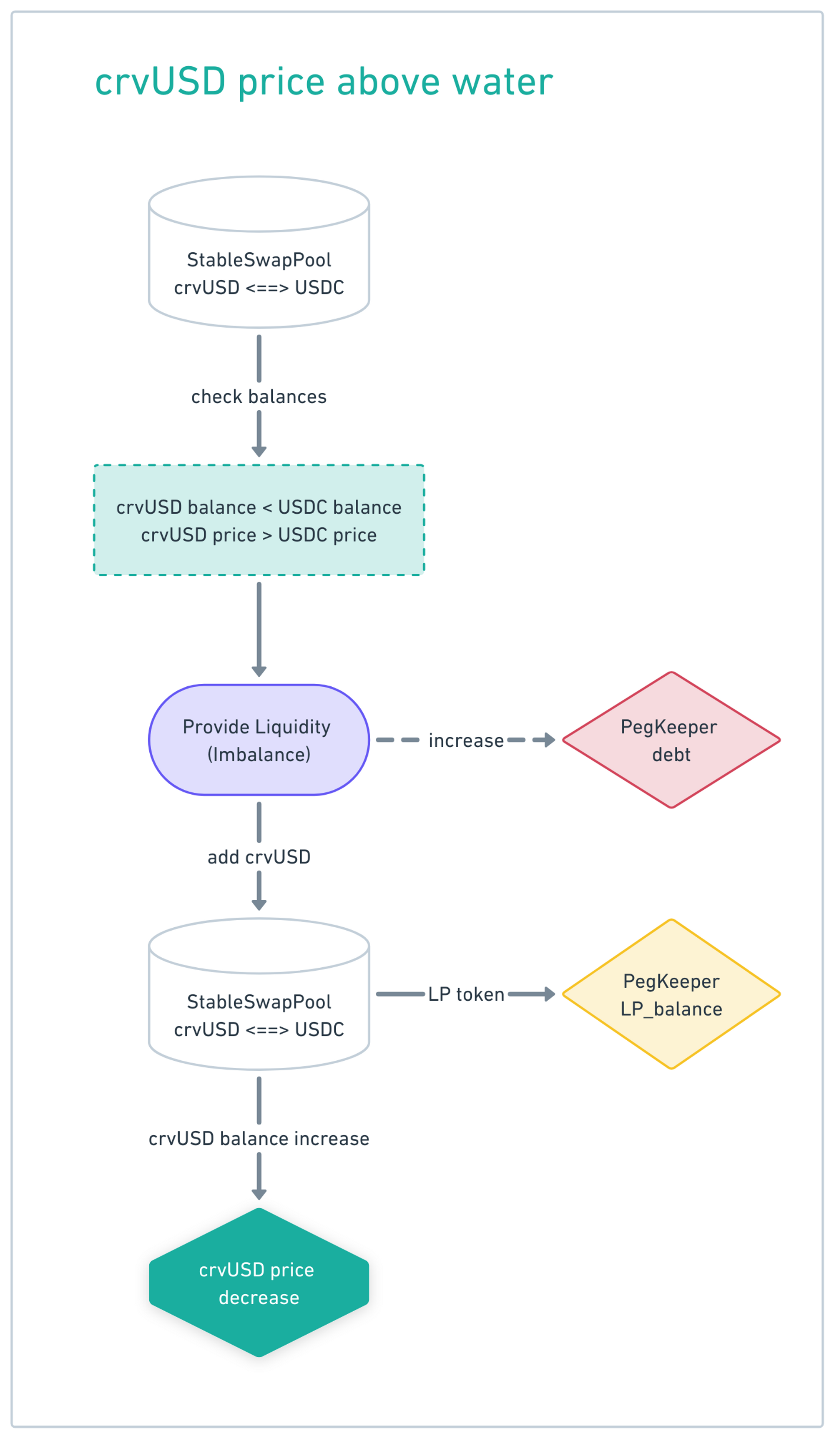When $P_{crvUSD}<1$, PegKeeper will withdraw crvUSD from crvUSD-USDC-StableSwapPool. After this, the debt of PegKeeper will decrease and crvUSD's price will gradually return.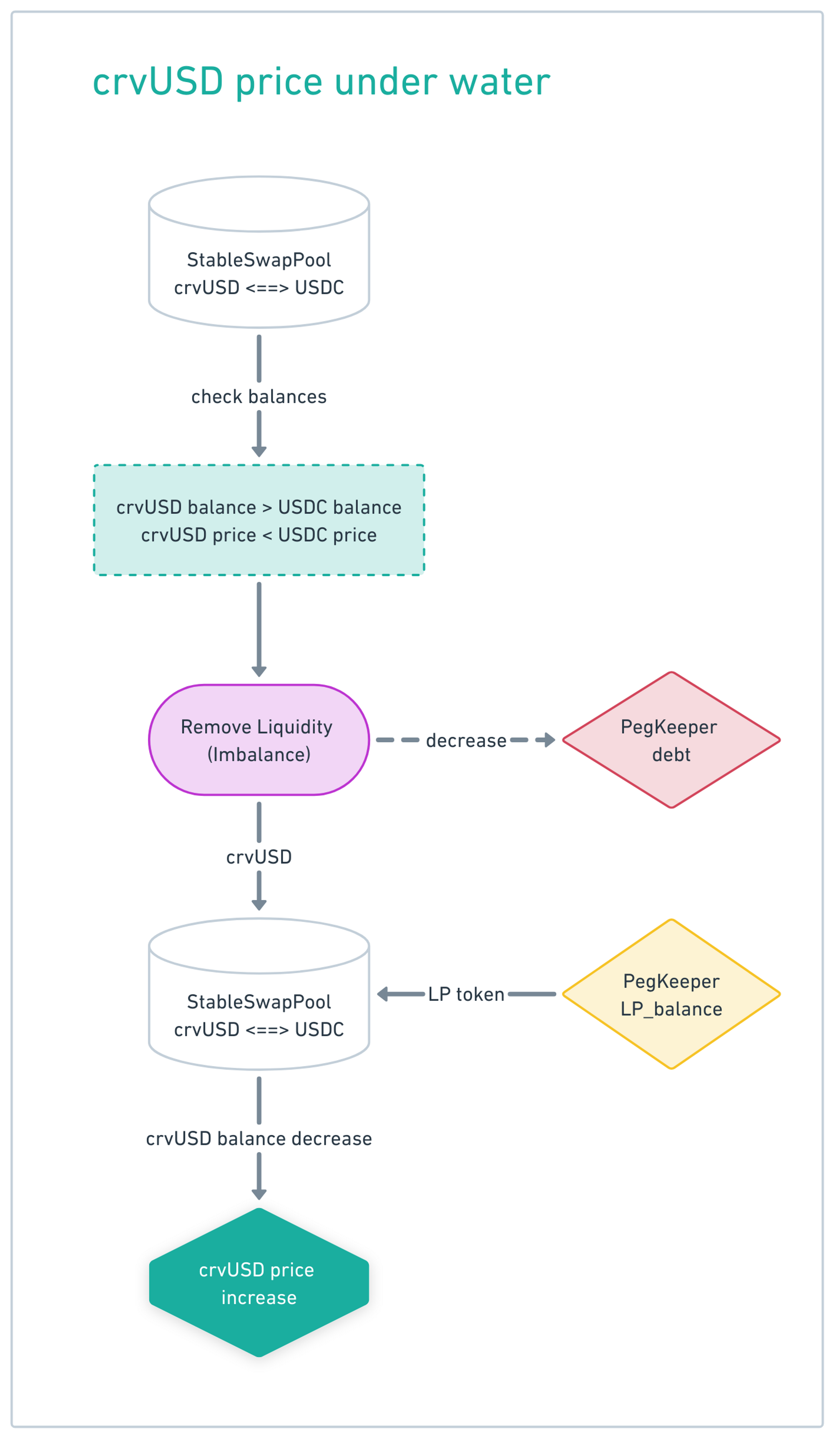Due to the high price of crvUSD during addition and the low price of crvUSD during removal, PegKeeper will have some LP tokens remaining after repaying the debt. These LP tokens represent the profit generated by PegKeeper.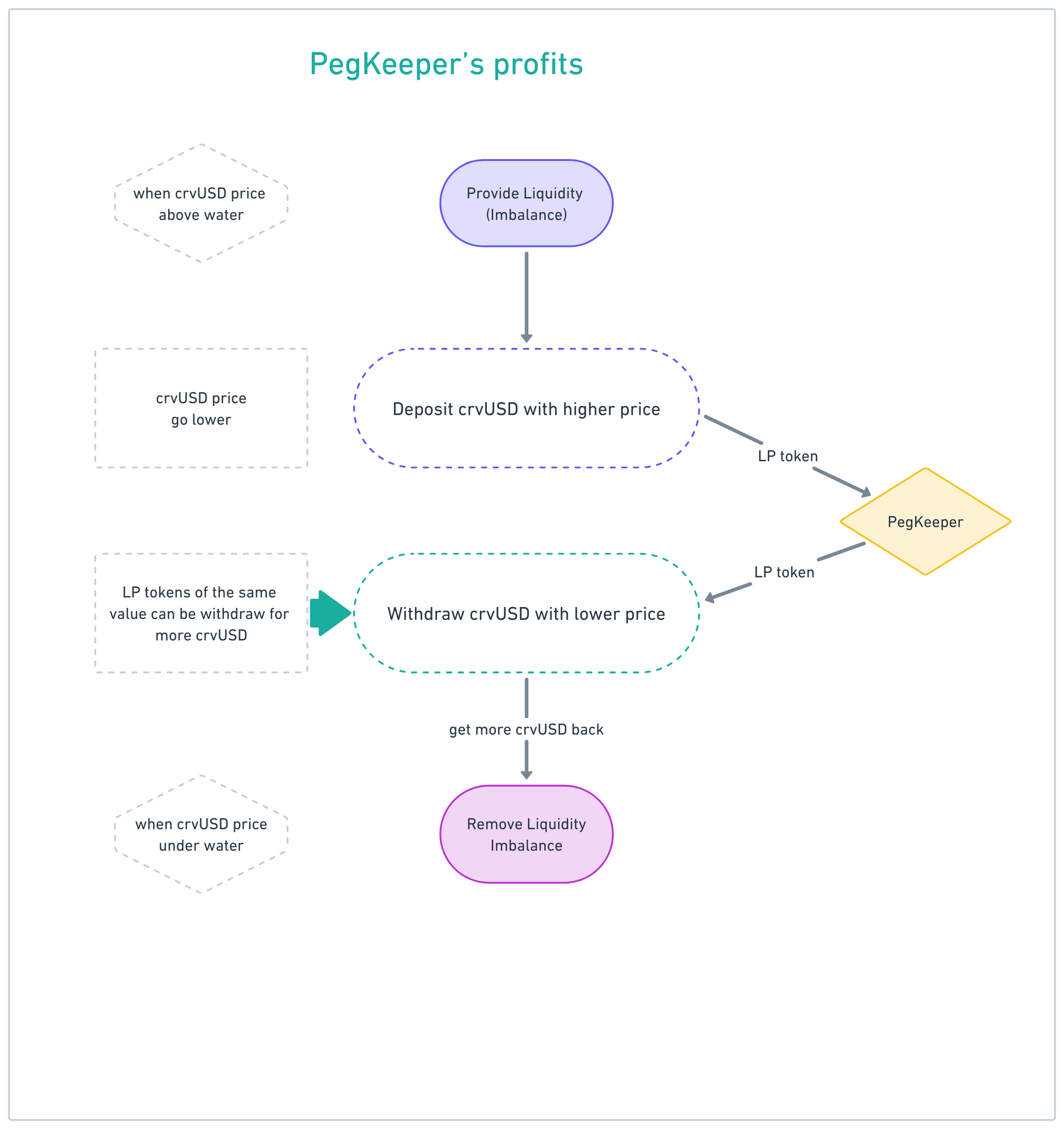## 5. Risks of liquidation and strategies

### 5.1 Risks

If your collateral enters soft-liquidation mode, you cannot partially withdraw or add more collateral to your position, which means you can only close your position. If the price of the collateral drops sharply over a short time interval, your position will be hard-liquidated, with no option of de-liquidation. Please choose your leverage wisely, as you would with any collateralized debt position.

Because arbitrageurs are doing soft-liquidation, the profit of the arbitrageurs can be seen as a loss for liquidity providers. When the external price falls into the soft-liquidation range and rises back up 100%, the user's assets do not rise back up 100%. This means there is unrecoverable loss during soft-liquidation.

### 5.2 Strategies

The Initial soft-liquidation price P_ISL and the corresponding band number is band_begin**.**

The end soft-liquidation price (P_FSL) and the corresponding band number is band_end

N is the band with which you choose in the advanced mode

$N=band\_end-band\_begin$

Band_begin:

$Band\_begin=log_{\frac{A}{A-1}}{(MAX\_crvUSD\_borrowable/debt)}$

The user's unrecoverable loss during soft-liquidation is mainly related to three aspects:

1. The smaller the band_begin, the easier it is to enter the soft-liquidation interval and trigger soft liquidation when the price drops.
2. As band_end increases, it is less likely to exit the soft-liquidation interval.
3. As N increases, each band has less liquidity, resulting in a less soft-liquidation unrecoverable ****loss per band.

A bigger band number means a lower price. Liquidation is done from smaller bands to bigger bands, while de-liquidation is done from bigger bands to smaller bands.

The choice of N and crvUSD borrow amount will affect LTV_max, band_begin and band_end.

Let's compare the consequences of selecting different N values.

N_small, [band_begin_N_small, band_end_N_small], LTV_max_N_small, Loss_N_small

versus

N_big, [band_begin_N_big, band_end_N_big], LTV_max_N_big, Loss_N_big

#### 5.2.1 Strategy A: Use a fixed amount of collateral to maximize borrowable crvUSD

When you deposit 1 wstETH into the pool and borrow crvUSD at the maximum possible amount, the larger the value of N, the smaller the value of MAX_crvUSD_borrow, and the larger the value of band_end, while band_begin remains unchanged.

eg: N=4, 2057-2141 & N=50, 1295-2141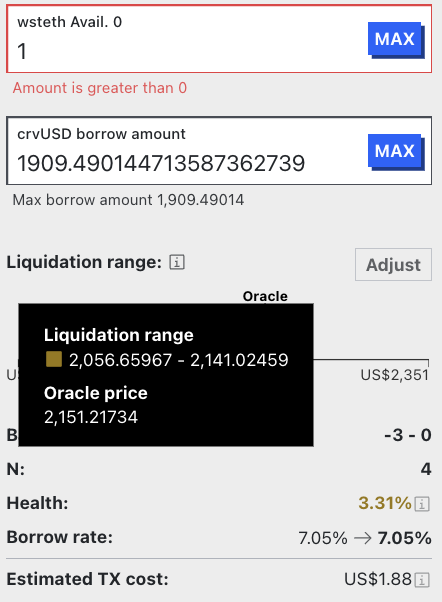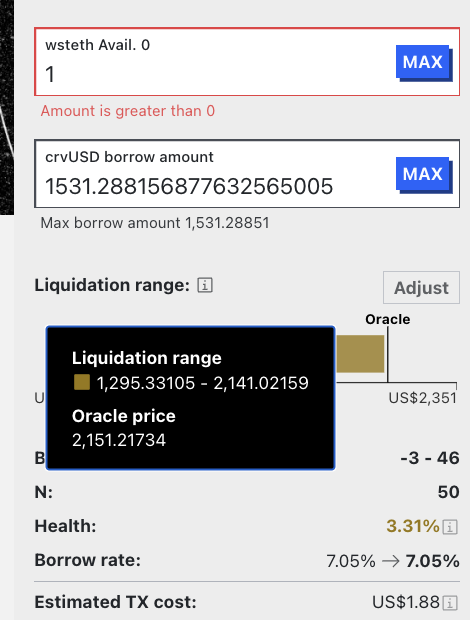When N is small, it will bring a larger band_end, which means a lower soft liquidation price. The band_begin is not related to the choice of A strategy and N. Under Strategy A, the size of N is not related to the possibility of entering the soft-liquidation interval (band_begin), but is related to leaving the soft-liquidation interval (band_end). When leaving the soft-liquidation interval, the liquidity will completely become crvUSD. When the price rebounds, re-entering the de-liquidation interval will bring losses to users.

Different strategies are adopted based on different expectations for future trends.

1. When the expected future price is expected to drop sharply beyond band_end_N_big and cannot rebound in the long term, adopting N_small will have a greater advantage (When N is large, the unrecoverable loss from a single full interval soft-liquidation is greater).

a. Choosing N_small makes it easier to exit the soft-liquidation interval, resulting in a smaller unrecoverable loss from a single full interval soft-liquidation.

b. Choosing N_small will result in a higher LTV_max.

2. When the expected future price is going to experience a cliff-like drop that exceeds band_end_N_small but not band_end_N_big, and it cannot rebound in the long term, taking N_small may have some advantages:

a. Choosing N_small makes it easier to exit the soft-liquidation interval. The unrecoverable loss from a single-time full interval soft-liquidation using N_small is generally not bigger than multiple-times unrecoverable loss using N_big.

b. Choosing N_small will result in a higher LTV_max.

3. When the expected future price is anticipated to decrease and not exceed band_end_N_small, using N_big will be more advantageous when fluctuating between band_begin_N_small and band_end_N_small, despite having a smaller LTV_max. During this time, the same soft-liquidation occurs in [band_begin_N_small, band_end_N_small] & [band_begin_N_big, band_end_N_big]. However, using N_big results in a smaller amount of liquidity being liquidated in each band compared to using N_small. As a result, using N_big significantly reduces unrecoverable loss during the soft-liquidation.

#### 5.2.2 Strategy B: Deposit a fixed amount of collateral and borrow a fixed amount of crvUSD

Choosing N_small has greater advantages. By using N_small, there will be a higher health score, and band_begin_N_small > band_begin_N_big, band_end_N_small < band_end_N_big, which means it is less likely to enter the soft-liquidation mode and more likely to exit the soft-liquidation interval.